# Geometric Mean Calculator

Created by Hanna Pamuła, PhD candidate
Reviewed by Bogna Szyk
Last updated: Apr 06, 2022

With this geometric mean calculator, all calculations will be a pleasure! Just type the numbers of which you want to calculate the geometric mean and the result will appear in no time. Remember that you may enter up to 30 numbers - extra boxes will appear if you fill in the previous ones. If you're wondering what is a geometric mean and you're looking for a definition and formula of the geometric mean, keep reading.

## What is a geometric mean? Geometric mean definition and formula

A geometric mean - with arithmetic, weighted and harmonic means - is one of the most useful and common type of averages. Geometric mean may be defined as:

n-th root of the product of n values

The n-th root is at the same time the power of one divided by n. Thus, the geometric mean definition may be stated as:

the product of the values to the power of 1/n

The general geometric mean formula looks then as follows:Simply speaking, if you are wondering how to find geometric mean, just multiply your values and take a square root (for two numbers), cube root (for three numbers), fourth root (for four numbers) etc.

Generally, the geometric mean applies only to positive numbers.

## Geometric mean vs arithmetic mean

In many cases the arithmetic mean is the average we're looking for. However, there are some cases when the geometric mean is the preferable mean:

• different numeric ranges - e.g., we want to take into account two values assuming they are equally important: one from 0-5 range and another from 900-1000 range. Change of the smaller number, even significant one (e.g. from 1 to 5) will result in tiny difference in arithmetic mean. The arithmetic mean neglects the smaller number, but that's the perfect case for geometric mean.
• the data is skewed downwards, has large positive outliers - geometric mean can take care of that, in contrast to the arithmetic average.

Have a look at the table to find direct comparison: geometric mean vs arithmetic mean, with definitions, formulas and applications of both types of averages:

Arithmetic Mean

Geometric Mean

Definition

sum of observations divided by the total number of observations

the 'n'th root product of 'n' observations

General formulaExamples:

4 and 9

A = (4 + 9) / 2 = 6.5

A = √(4 * 9) = √36 = 6

4 and 900

A = (4 + 900) / 2 = 452

A = √(4 * 900) = √3600 = 60

Applications

many different fields, e.g. economics, biology, history, everyday life

business (investment, growth, CAGR), math (rectangle area in terms of square side, analogically volume), image and signal processing (spectral flatness, filtering, choosing aspect ratio)

Relationship

Arithmetic mean ≥ Geometric mean (for non-negative data)

Also, the relationship between those two means may be defined:

the logarithm of the geometric mean of the set of numbers is the arithmetic mean of the logs of these numbers.

For example, for three numbers:

log((a * b * c)^(1/3)) = (1/3) * log(a * b * c) = (1/3) * (log(a) + log(b) + log(c))

In other words, you can find the geometric mean by:

1. Taking the logarithms of your data
2. Finding the arithmetic mean (often simply called average)
3. Taking the antilog of the result (remember that the antilogarithm is simply the exponentiation).

## Geometric mean triangles and other applications in geometry

Geometric mean theorem (also called right triangle altitude theorem) states that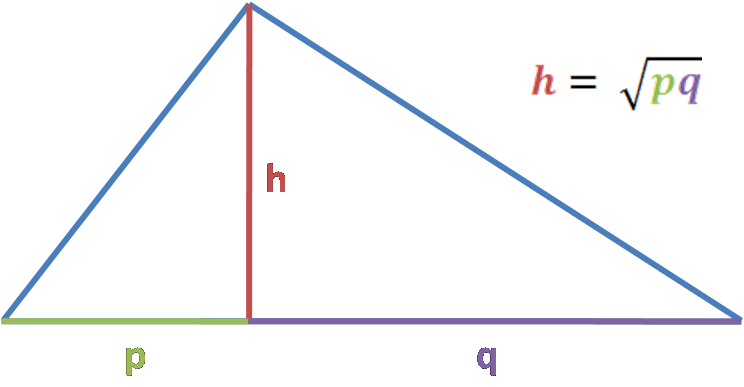Geometric mean of the two segments of a hypotenuse equals the altitude of a right triangle from its right angle

h = √(p * q)

Let's have a look at geometric mean triangles and proof of this theorem. We'll show that in two ways - using similarity of the triangles and Pythagorean theorem.

Following the image description, h is the altitude of a right triangle from its right angle, which splits the hypotenuse into two segments: p and q.

1. Triangles similarity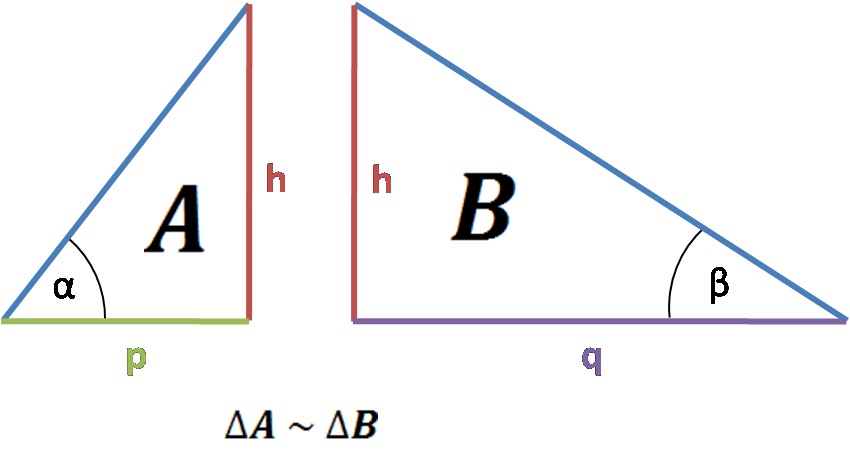• A hypotenuse is splitting the right triangle into two smaller triangles, let's call them triangle A and B. Triangle A is similar to our base triangle (two angles in common, 90° and α), and triangle B is also similar to a base triangle (congruent 90° and β). From that, we can imply that triangle A is similar to triangle B.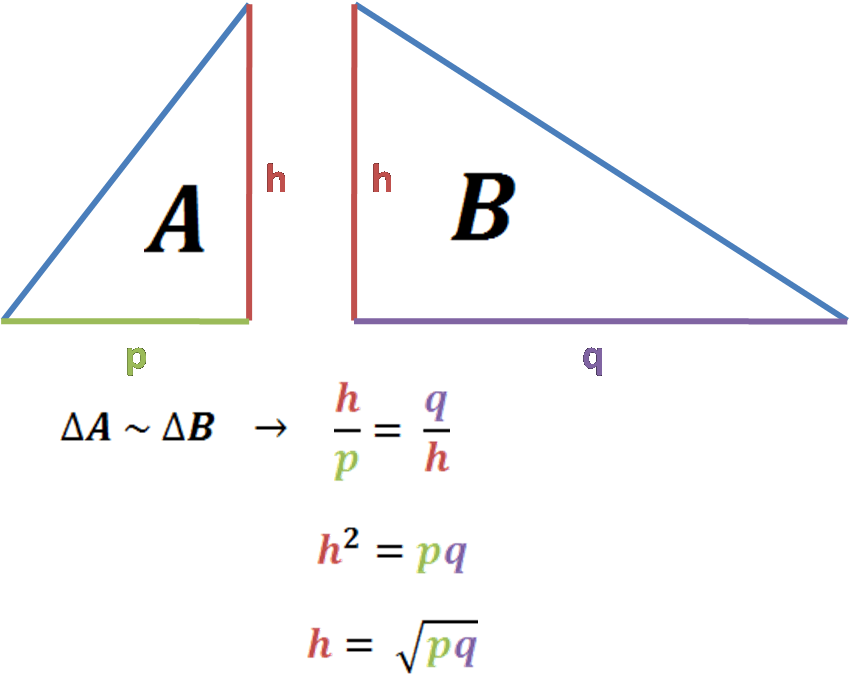• If the triangles are similar, we can write that the ratio between their congruent sides is the same. So e.g. longer leg of a triangle A (h) to shorter leg of that triangle (p) equals triangle's B longer leg to shorter leg (q / h).

• After simplifications, we're coming to the final formula:

h = √(p * q)

If you're not sure how we did the ratios here to arrive at this result, check out our ratio calculator!

1. Pythagorean theorem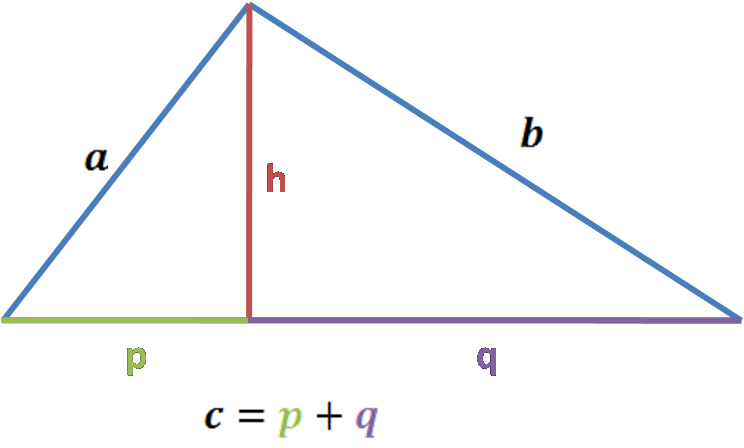Knowing the Pythagorean theorem, we can write equations for three right triangles: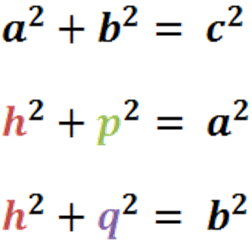Then, we can put altitude onto one side and add the last two equations: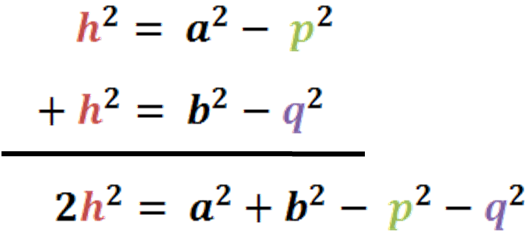After simplifications, we get to the final result: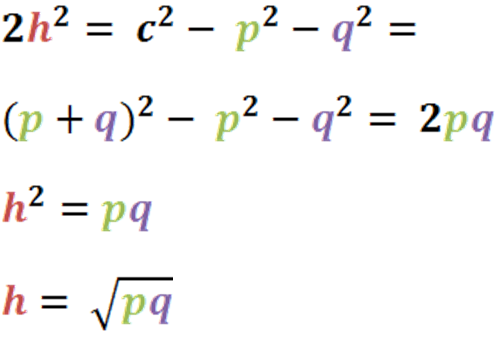It was a piece of cake, wasn't it?

The geometric mean is also applied in other geometric shapes and problems:

• There are many geometric mean relationships in ellipsis - e.g. taking the geometric mean of the maximum and minimum distances of the ellipse from a focus, you'll get the semi-minor axis.
• Geometric mean of the distance to the closest and furthest point of a sphere is the distance to the horizon of a sphere.
• Looking for the approximate derivation of , you'll also find the geometric mean formula essential.

## How to find a geometric mean? An example on how to use geometric mean calculator

Let's take an easy example. Assume we want to know what is the geometric mean of 7 and 12:

1. Type the first value into the box It's 7 in our case.
2. Enter next values. For us, it's 12 only. If you have more numbers than two, keep typing the values into separate boxes. Only eight are shown, but you can enter up to 30 numbers - they'll appear when the time comes.
3. Here you go! The geometric mean calculator displays the geometric mean on the go! Thanks to the tool, we found out that the geometric mean of 2 typed numbers is 9.1652. Great!

## Means in everyday life

In this article we specifically explained one type of a mean - geometric mean. But means are all around us! We've chosen only a couple examples of our calculators which are using averaging, but we're sure you could find more applications than that.

1. Health

• max heart rate calculator is finding your average heart rate to which you should aim during a training
• mean arterial pressure is calculating the average arterial blood pressure during a single cardiac cycle
• child height is helping to find out how tall your children can be, basing on formulas using the average of parents height

2. Physics and Statistics

3. Everyday life

• average rating may be useful to calculate the average rating based on 5-option voting
• pace calculator can help to estimate the speed at which someone or something moves - like calculation of average running or swimming speed

Can you think of other applications of an average?

Hanna Pamuła, PhD candidate
Values (you may enter up to 30 numbers)
#1
#2
#3
#4
#5
#6
#7
#8
People also viewed…

### Black Hole Collision Calculator

The Black Hole Collision Calculator lets you see the effects of a black hole collision, as well as revealing some of the mysteries of black holes, come on in and enjoy!

### Car vs. Bike

Everyone knows that biking is awesome, but only this Car vs. Bike Calculator turns biking hours into trees! 🌳

### SAS triangle

You can calculate every quantity in a triangle just by giving us two sides and an angle: try our SAS triangle calculator!

### Square inches of a circle

Our square inches of a circle calculator helps you to calculate the area of a circle in inches.